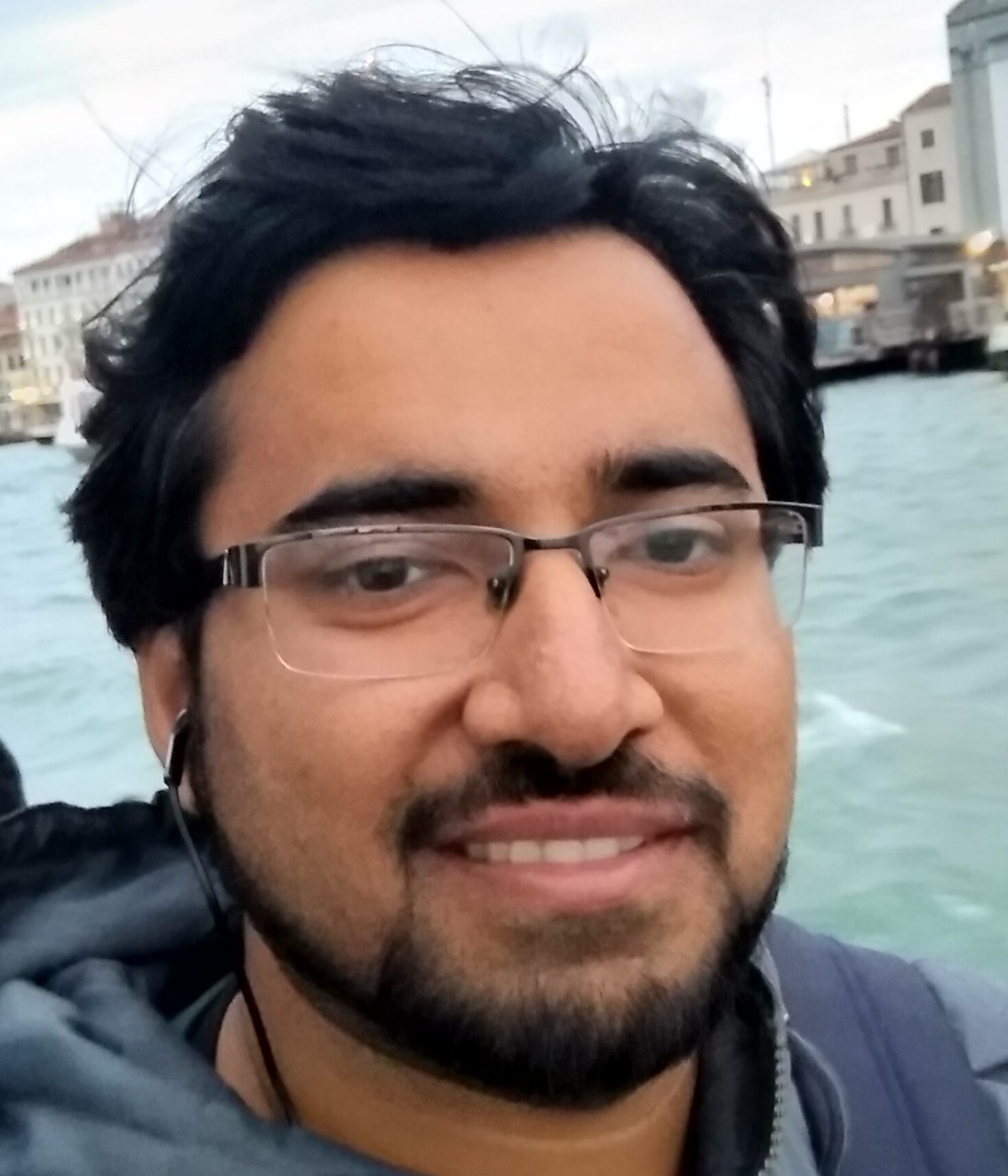# BARC talk by Shahbaz Khan - CANCELLED DUE TO COVID-19

Wednesday, 25 March 2020, Shahbaz Khan, Postdoc at the University of Helsinki, will give a talk on "Incremental DFS algorithms: a theoretical and experimental study".

Title:
Incremental DFS algorithms: a theoretical and experimental study

Abstract:
The depth first search (DFS) tree is a fundamental data structure used for solving various graph problems. For a given graph G = (V, E) on n vertices and m edges, a DFS tree can be built in O(m + n) time. In the last 20 years, a few algorithms have been designed for maintaining a DFS tree efficiently under insertion of edges. For undirected graphs, there are two prominent algorithms, namely, ADFS1 and ADFS2 [ICALP14] that achieve total update time of  and O(n2) respectively. For directed acyclic graphs, the only non-trivial algorithm, namely, FDFS [IPL97] requires total O(mn) update time. However, even after 20 years of this result, there does not exist any non-trivial incremental algorithm for maintaining a DFS tree in directed graphs with o(m2) worst case bound.
In this paper, we carry out extensive experimental and theoretical evaluation of the existing incremental DFS algorithms in random graphs and real world graphs and derive the following results.

1.
For insertion of a uniformly random sequence of  edges, each of ADFS1, ADFS2 and FDFS perform equally well and are found to take Θ(n2) time experimentally. This is quite surprising because the worst case bounds of ADFS1 and FDFS are greater than Θ(n2) by a factor of  and m/n respectively, which are also proven to be tight. We complement this experimental result with a probabilistic analysis of these algorithms establishing Õ(n2) bound on their time complexity. For this purpose, we derive results about the structure of a DFS tree in a random graph. These results are of independent interest in the domain of random graphs.

2.
The insight that we developed about DFS tree in random graphs leads us to design an extremely simple algorithm for incremental DFS that works for both undirected and directed graphs. Moreover, this algorithm theoretically matches and experimentally outperforms the state-of-the-art algorithm in dense random graphs. Furthermore, it can also be used as a single-pass semi-streaming algorithm for computing incremental DFS and strong connectivity for random graphs using O(n log n) space.

3.
Even for real world graphs, which are usually sparse, both ADFS1 and FDFS turn out to be much better than their theoretical bounds. Here again, we present two simple algorithms for incremental DFS for directed and undirected graphs respectively, which perform very well on real graphs. In fact our proposed algorithm for directed graphs almost always matches the performance of FDFS.

Bio:Shahbaz is a postdoc at University of Helsinki and had previously worked as a postdoc in University of Vienna. He completed his PhD under Prof. Surender Baswana at Indian Institute of Technology Kanpur. His research have been mainly on dynamic graph algorithms for classical graph problems wherein he looked at it from both theoretical and experimental perspectives.